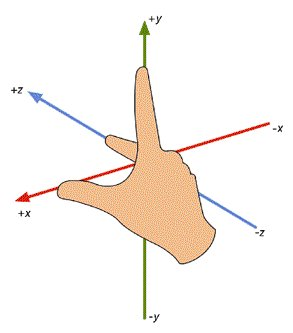# # Vector3

## # 描述

MC中使用的是右手坐标系，如下图所示。后文中的上下前后左右均是以steve面向z轴正方向得出来的。MC中东西方向为X坐标轴，其中X轴正方向为东，X轴负方向为西；南北方向为Z坐标轴，其中Z轴正方向为南，Z轴负方向为北。即左西右东前南后北。## # 构造函数

### # Vector3(x, y, z)

• 描述

用于构造一个向量或者3维点坐标。

• 参数

参数名 数据类型 说明
x float 向量的x分量
y float 向量的y分量
z float 向量的z分量
• 返回值

数据类型 说明
Vector3 返回Vector3(x, y, z)
• 示例

``````from common.utils.mcmath import Vector3
newOne = Vector3(1, 2, 3)
``````

### # Vector3(vecTuple)

• 描述

用于构造一个向量或者3维点坐标。

• 参数

参数名 数据类型 说明
vecTuple tuple(float, float, float) 长度为3的tuple数组
• 返回值

数据类型 说明
Vector3 返回Vector3(vecTuple, vecTuple, vecTuple)
• 示例

``````from common.utils.mcmath import Vector3
import client.extraClientApi as clientApi
comp = clientApi.CreateComponent(entityId, "Minecraft", "pos")
entityFootPos = comp.GetFootPos() # 通过位置组件获取实体位置
posVec = Vector3(entityFootPos) # 直接将该位置tuple转换成Vector3以便后续计算
``````

## # 静态方法

### # One

• 描述

用于编写 Vector3(1, 1, 1) 的简便方法。

• 返回值

数据类型 说明
Vector3 返回Vector3(1, 1, 1)
• 示例

``````from common.utils.mcmath import Vector3
newOne = Vector3.One()
``````

### # Up

• 描述

用于编写 Vector3(0, 1, 0) 的简便方法。

• 返回值

数据类型 说明
Vector3 返回Vector3(0, 1, 0)
• 示例

``````from common.utils.mcmath import Vector3
newOne = Vector3.Up()
``````

### # Down

• 描述

用于编写 Vector3(0, -1, 0) 的简便方法。

• 返回值

数据类型 说明
Vector3 返回Vector3(0, -1, 0)
• 示例

``````from common.utils.mcmath import Vector3
newOne = Vector3.Down()
``````

### # Left

• 描述

用于编写 Vector3(-1, 0, 0) 的简便方法，对应MC中的西面。

• 返回值

数据类型 说明
Vector3 返回Vector3(-1, 0, 0)
• 示例

``````from common.utils.mcmath import Vector3
newOne = Vector3.Left()
``````
• 描述

用于编写 Vector3(1, 0, 0) 的简便方法，对应MC中的东面。

• 返回值

数据类型 说明
Vector3 返回Vector3(1, 0, 0)
• 示例

``````from common.utils.mcmath import Vector3
newOne = Vector3.Right()
``````

### # Forward

• 描述

用于编写 Vector3(0, 0, 1) 的简便方法，对应MC中的南面。

• 返回值

数据类型 说明
Vector3 返回Vector3(0, 0, 1)
• 示例

``````from common.utils.mcmath import Vector3
newOne = Vector3.Forward()
``````

### # Backward

• 描述

用于编写 Vector3(0, 0, -1) 的简便方法，对应MC中的北面。

• 返回值

数据类型 说明
Vector3 返回Vector3(0, 0, -1)
• 示例

``````from common.utils.mcmath import Vector3
newOne = Vector3.Backward()
``````

### # Dot

• 描述

两个向量的点积。

点积是一个浮点值，它等于 将两个向量的大小相乘，然后乘以向量之间角度的余弦值。

对于 normalized 向量，如果它们指向完全相同的方向，Dot 返回 1； 如果它们指向完全相反的方向，返回 -1；如果向量彼此垂直，则 Dot 返回 0。

• 参数

参数名 数据类型 说明
a Vector3 向量a
b Vector3 向量b
• 返回值

数据类型 说明
float 两个向量的点积
• 示例

``````from common.utils.mcmath import Vector3
a = Vector3(1, 2, 3)
b = Vector3(0, 3, 1)
c = Vector3.Dot(a, b) # 1 * 0 + 2 * 3 + 3 * 1 = 9
``````

### # Cross

• 描述

两个向量的叉积。

两个向量的叉积生成第三个向量， 该向量垂直于两个输入向量。结果的大小等于： 将两个输入的大小相乘，然后乘以输入之间角度的正弦值。 可以使用“右手定则”确定结果向量的方向。用右手的四指先表示向量a的方向，然后手指朝着手心的方向摆动到向量b的方向，大拇指所指的方向就是向量c的方向。

• 参数

参数名 数据类型 说明
a Vector3 向量a
b Vector3 向量b
• 返回值

数据类型 说明
float 两个向量的点积
• 示例

``````from common.utils.mcmath import Vector3
a = Vector3(1, 2, 3)
b = Vector3(0, 3, 1)
c = Vector3.Cross(a, b)
``````

## # 成员方法

### # Length

• 描述

返回该向量的长度。

向量长度为 `(x*x+y*y+z*z)` 的平方根。

如果只需要比较一些向量的大小， 则可以使用LengthSquared()函数比较它们的平方数（计算平方数更快）。

• 返回值

数据类型 说明
float 该向量长度的平方
• 示例

``````from common.utils.mcmath import Vector3
a = Vector3(3, 4, 0)
print a.Length() # 打印 5.0
``````

### # LengthSquared

• 描述

返回该向量的长度的平方。

• 返回值

数据类型 说明
float 该向量标准化后的向量
• 示例

``````from common.utils.mcmath import Vector3
a = Vector3(3, 4, 0)
print a.LengthSquared() # 打印 25.0
``````

### # ToTuple

• 描述

返回该向量的tuple形式(x, y, z)，便于玩家转换后作为其他事件的参数进行传递。

• 返回值

数据类型 说明
tuple 返回该向量的tuple形式(x, y, z)
• 示例

``````from common.utils.mcmath import Vector3
a = Vector3(3, 4, 0)
print a.ToTuple() # 打印 (3, 4, 0)
``````

### # Normalized

• 描述

返回长度为 1 时的该向量。

进行标准化时，向量方向保持不变，但其长度为 1.0。

请注意，当前向量保持不变，返回一个新的归一化向量。如果 要归一化当前向量，请使用Normalize函数。

如果向量太小而无法标准化，则返回零向量。

• 返回值

数据类型 说明
Vector3 该向量标准化后的向量
• 示例

``````from common.utils.mcmath import Vector3
a = Vector3(3, 4, 0)
print a.Normalized() # 打印结果(0.6, 0.8, 0)
print a # 打印结果(3, 4, 0)，a并没有发生变化
``````

### # Normalize

• 描述

使该向量标准化，向量方向保持不变，但其长度变为 1.0。

请注意，该函数无返回值，仅改变当前向量，如果要返回当前向量的标准化值且不改变该向量，请使用Normalized函数。

如果向量太小而无法标准化，则设置为零向量。

• 示例

``````from common.utils.mcmath import Vector3
a = Vector3(3, 4, 0)
a.Normalize()
print a # 打印结果(0.6, 0.8, 0)，a被标准化
``````

### # Set

• 描述

设置该向量的值

• 参数

参数名 数据类型 说明
x float 向量的x分量
y float 向量的y分量
z float 向量的z分量
• 示例

``````from common.utils.mcmath import Vector3
a = Vector3(3, 4, 0)
a.Set(1, 2, 3)
print a # 打印结果(1, 2, 3)
``````

## # 成员变量

• 描述

可以直接通过`.`运算符访问向量的x、y、z分量。也可以通过`[]`直接获取，0、1、2分别对应x、y、z。但不能直接通过该方法对各个分量进行设置，只能通过Set方法来进行设置。

• 示例

``````from common.utils.mcmath import Vector3
a = Vector3(3, 4, 5)
print a.x # 3
print a.y # 4
print a.z # 5
print a # 3
print a # 4
print a # 5
``````

## # 运算符

### # operate +

• 描述

向量加法，两向量相加等于各分量之和。向量与常数相加等于各分量分别加上该常数。

• 示例

``````from common.utils.mcmath import Vector3
a = Vector3(3, 4, 0)
b = Vector3.One()
print a + 5 # 打印结果(8, 9, 5)
print a + b # 打印结果(4, 5, 1)
``````

### # operate -

• 描述

向量减法，两向量相加等于各分量之差。向量与常数相减等于各分量分别与该常数求差。

向量求反，返回相反方向的向量

• 示例

``````from common.utils.mcmath import Vector3
a = Vector3(3, 4, 0)
b = Vector3.One()
print a - 2 # 打印结果(1, 2, -2)
print 2 - a # 打印结果(-1, -2, 2)
print a - b # 打印结果(2, 3, -1)
print -a # 打印结果(-3, -4, 0)
``````

### # operate *

• 描述

向量乘法，两向量相乘等于各分量相乘相加，即向量点积，等价于Vector3.Dot(a, b)。向量与常数相乘等于各分量分别乘以该常数。

• 示例

``````from common.utils.mcmath import Vector3
a = Vector3(3, 4, 0)
b = Vector3.Up()
print a * 2 # 打印结果(6, 8, 0)
print a * b # 打印结果4
``````

### # operate /

• 描述

向量除法，仅支持向量与常数相除，等于各分量分别除以该常数。

• 示例

``````from common.utils.mcmath import Vector3
a = Vector3(3, 4, 0)
b = Vector3.One()
print a / 2 # 打印结果(1.5, 2, 0)
``````

### # operate ==

• 描述

判断两个向量是否相等，当各分量均相等时返回True

### # operate !=

• 描述

判断两个向量是否不等，当各分量均相等时返回False